Courses

# Test: Coordination Compounds 2 - From Past 28 Years Questions

## 30 Questions MCQ Test Chemistry Class 12 | Test: Coordination Compounds 2 - From Past 28 Years Questions

Description
This mock test of Test: Coordination Compounds 2 - From Past 28 Years Questions for Class 12 helps you for every Class 12 entrance exam. This contains 30 Multiple Choice Questions for Class 12 Test: Coordination Compounds 2 - From Past 28 Years Questions (mcq) to study with solutions a complete question bank. The solved questions answers in this Test: Coordination Compounds 2 - From Past 28 Years Questions quiz give you a good mix of easy questions and tough questions. Class 12 students definitely take this Test: Coordination Compounds 2 - From Past 28 Years Questions exercise for a better result in the exam. You can find other Test: Coordination Compounds 2 - From Past 28 Years Questions extra questions, long questions & short questions for Class 12 on EduRev as well by searching above.
QUESTION: 1

### An example of double salt is 

Solution:

Potash alum K2SO4 .Al2 (SO4)3 24H2O is a double salt.

QUESTION: 2

### The complex ion [Co(NH3)6]3+ is formed by sp3d2 hybridisation. Hence the ion should possess

Solution:

According to VSEPR theory, a molecule with six bond pairs must be octahedral.

QUESTION: 3

### K3[Al(C2O4)3] is called 

Solution:

IUPAC name is potas sium trioxalatoaluminate (III).

QUESTION: 4

Among the following complexes, optical activity is possible in 

Solution:

It is optically active when two Cl atoms are in cis position.

QUESTION: 5

Which of the following is common donor atom in ligands? 

Solution:

In the formation of a coordinate bond, the ligands donate a pair of electrons to the metal atom. Futher nitrogen and oxygen has great tendency to donate the pair of elctrons in most of  the compounds. Therefore both nitrogen and oxygen are common donor atoms in ligands.

QUESTION: 6

Among the following, the compound that is both paramagnetic and coloured, is 

Solution:

Among the following, the compound that is both paramagnetic and coloured, is 

QUESTION: 7

Which of the following may be considered to be an organometallic compound? 

Solution:

Organometallic compounds are the ones having a direct bond between a metal and carbon atoms. So, nickel carbonyl is one such compound.

QUESTION: 8

The number of geometrical isomers for [Pt (NH3)2 Cl2] is

Solution:

Pt (NH3)2 Cl2 is a disubstituted complex and shows only cis-& trans-isomers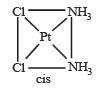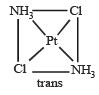QUESTION: 9

The formula for the complex, dichlorobis (urea) copper (II) is 

Solution: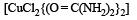QUESTION: 10

Which of the following statements is correct ? (Atomic number of Ni = 28) 

Solution: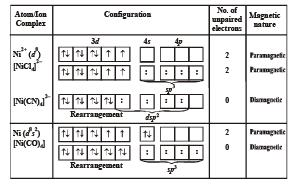QUESTION: 11

The number of geometrical isomers of the complex [Co(NO2)3 (NH3)3] is 

Solution:

[Co(NO2)3 (NH3)3]

Possible geometrical isomers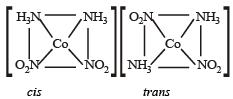Total geometrical isomers = 2

QUESTION: 12

The total number of possible isomers for the complex compound [CuII (NH3)4] [PtII Cl4] 

Solution:

The total number of isomers for the complex compound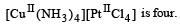These four isomers are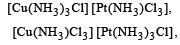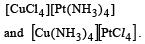The isomer [Cu (NH3)2 Cl2][Pt (NH3)2 Cl2] does not exist due to both parts being neutral.

QUESTION: 13

IUPAC name of [Pt(NH3)3 (Br) (NO2) Cl] Cl is 

Solution:

We know that IUPAC name of [Pt (NH3)3 (Br) (NO2)Cl]Cl is triamminebromochloronitroplatinum (IV) chloride.

QUESTION: 14

A co-ordination complex compound of cobalt has the molecular formula containing five ammonia molecules, one nitro group and two chlorine atoms for one cobalt atom. One mole of this compound produces three mole ions in an aqueous solution. On reacting this solution with excess of AgNO3 solution, we get two moles of AgCl precipitate. The ionic formula for this complex would be 

Solution:

As it forms two moles of silver chloride thus it has two moles of ionisable Cl.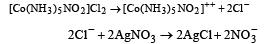QUESTION: 15

In which of the following compounds does iron exhibit zero oxidation state? 

Solution:

In [Fe(CO)5] Iron exist in zero oxidation state

QUESTION: 16

Which one of the following will show paramagnetism corresponding to 2 unpaired electrons?(Atomic numbers : Ni = 28, Fe = 26) 

Solution:

As in [NiCl4]–2 Chloride ion being a weak ligand is not able to paired the electron in d orbital.

QUESTION: 17

The number of unpaired electrons in the complex [Cr(NH3)6]Br3 is (Atomic number Cr = 24)

Solution:

In [Cr (NH3)6]Br3, Cr is in +3 oxidation state etc.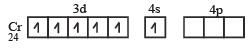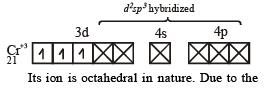presence of three unpaired electrons it is paramagnetic.

QUESTION: 18

Which one of the following complexes will have four different isomers ? 

Solution:

Complex Co(en)(NH3)2Cl2]Cl will have four different isomers.

(i) Geometrical isomers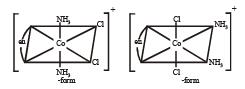(ii) Optical isomers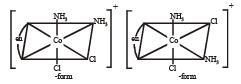QUESTION: 19

Which of the following will give maximum number of isomers? 

Solution:

[Cr(SCN)2 ( NH3) 4]+ shows linkage , geometrical and optical isomerism. Hence produces maximum no. of isomers.

QUESTION: 20

Which of the following will exhibit maximum ionic conductivity? 

Solution:

The complex ions which give maximum ion in solution exhibit maximum ionic conductivity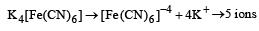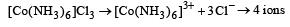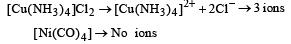QUESTION: 21

Which statement is incorrect? 

Solution:

(CO) carbonyl group being a strong ligand paired all electrons present in d orbital of Ni.
Hence form dsp2 hybrid orbitals and hence the shape of molecule is square planar.
In Ni(CO)4 complex, Ni will have 3d10 configuration. It has tetrahedral geometry but diamagnetic as there are no unpaired electrons.

QUESTION: 22

Which of the following organometallic compound is σ and π bonded? 

Solution:

[Co(CO) 5 NH3]2+ . In this complex. Coatom attached with NH3 through σ bonding with CO attached with dative π-bond.

QUESTION: 23

Oxidation number of Ni in [Ni(C2O4)3]4– is 

Solution: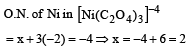QUESTION: 24

Atomic number of Cr and Fe are respectively 24 and 26, which of the following is paramagnetic? 

Solution:

Cr3+ has 4sº 3d3 electronic configuration with 3 unpaired electrons, hence paramagnetic.
In other cases pairing of d-electrons take place in presence of strong field ligands such as CO or CN.
In Cr(CO)6 molecule 12 electrons are contributed by CO group and it contain no odd electron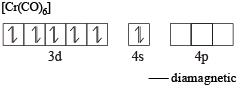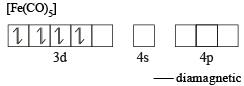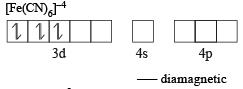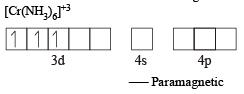QUESTION: 25

The hypothetical complex chlorodiaquotriammine cobalt (III) chloride can be represented as 

Solution:

Chloro diaquatriammine cobalt (III) chloride is [CoCl(NH3)3(H2O)2]Cl2

QUESTION: 26

CuSO4 when reacts with KCN forms CuCN, which is insoluble in water. It is soluble in excess of KCN due to formation of the following complex 

Solution:

Copper sulphate react with KCN to give white ppt of Cu(CN)2 and cyanogen gas. The insoluble copper cynaide dissolve in excess of KCN and give soluble potassium cuprocyanide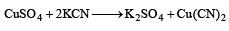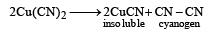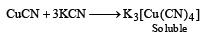QUESTION: 27

Which one of the following octahedral complexes will not show geometric isomerism? ( A and B are monodentate ligands) 

Solution:

MA3 B3 – 2 geometrical isomers
MA2 B4 – 2 geometrical isomers
MA4 B2 – 2 geometrical isomers
The complexes of general formula Ma6 and Ma5b octahedral geometry do not show geometrical isomerism.

QUESTION: 28

According to IUPAC nomenclature sodium nitroprusside is named as 

Solution:

IUPAC name of sodiumnitroprusside Na2[Fe(CN)5NO] is sodium pentacyanonitrosoyl ferrate (III) because in it NO is neutral ligand.
Hence 2×O.N. of Na + O.N. of Fe + 5×O.N. of CN       1×O.N. of NO = 02×(+1) + O.N. of Fe + 5 ×(–1) +1×0 = 0
O.N. of Fe = 5 – 2 = +3,
Hence ferrate (III)

QUESTION: 29

The number of unpaired electrons in the complex ion [CoF6]3– is (Atomic no.: Co = 27) 

Solution:

Cohere is in +3 oxidation state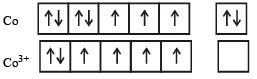Unpaired electrons  = 4 and sp3d2 hybridisation and octahedral shape.

QUESTION: 30

Among the following, which is not the π-bonded organometallic compound? 

Solution:

π bonded organometallic compounds is indicated by the greek latter 'η' with a number.
The prefixes η2, η5 and η6 indicate that 2, 5 and 6 carbon atom are bound to the metal in the compound.(CH3)4Sn does not involve any pi (π) bond formation. This is a π bonded organometallic compound